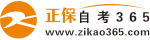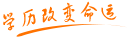#24小时客服：010-82335555# 自考“C语言程序设计”模拟试题十三

2007年05月29日    来源：   字体：   打印

一、单项选择题（每题1分，共20分）

1. C程序的基本单位是：（     ）

A.子程序    B.程序    C.子过程    D.函数

2.在C语言中，非法的八进制是：（     ）

A.016    B.018    C.017    D.02

3. 不是C语言实型常量的是：（     ）

A.55.0    B.0.0    C.55.5    D.55e2.5

4 .字符串“xyzw”在内存中占用的字节数是：（     ）

A.6    B.5    C.4    D.3

5. 若已定义f，g为double类型，则表达式：f=1，g=f+5/4的值是：（     ）

A.2.0    B.2.25    C.2.1    D.1.5   D.1.5   D.1.5   D.1.5

6. 若有语句char  c1=`d`，c2=`g`；printf（“%c，%d\n”，c2-`a`，c2-c1）；则输出结果为：（  ）

（a的ASCII码值为97）

A.M，2    B.G，3    C.G，2    D.D，g

7. 使用语句scanf（“a=%f，b=%d”，&a，&b）；输入数据时，正确的数据输入是：（     ）

A.a=2.2，b=3    B.a=2.2 b=3    C.2.2 3    D.2.2，3

8.表示关系12<=x<=y的C语言表达式为：（     ）

A.（12<=x）&（x<=y）  B. （12<=x）&&（x<=y）  C. （12<=x）|（x<=y）

D.（12<=x）||（x<=y）

9.设x=1，y=2，m=4，n=3，则表达式x>y？x：m<n？y：n的值为：（     ）

A.1    B.3    C.2    D.4

10. 若有说明和语句：int a=5，b=6；b*=a+1；则b的值为：（     ）

A.5    B.6    C.31    D.36

11. 设整型变量s，t，c1，c2，c3，c4的值均为2，则执行语句（s=c1==c2）||（t=c3>c4）后，s，t的值为：（     ）

A.1，2   B.1，1    C.0，1    D.1，0

12. 语句for（a=0，b=0；b！=100&&a<5；a++）scanf（“%d”，&b）； scanf最多可执行次数为：（     ）

A.4    B.6    C.5    D.1

13. 对于for（s=2；  ；s++）可以理解为：（     ）

A. for（s=2；0 ；s++）      B. for（s=2；1 ；s++）

C. for（s=2；s<2 ；s++）    D. for（s=2；s>2；s++）

14. 若有char h=`a`，g=`f`；int a={1，2，3，4，5，6}；则数值为4的表达式为：（     ）

A.a[g-h]    B.a    C.a[`d`-`h`]    D.a[`h`-`c`]

15. 设：char s={“october”}；则printf（“%d\n”，strlen（s））；输出是：（  ）

A.7    B.8    C.10   D.11

16. 若有int a={2，2}，{2，6}，{2，6，2}}，则数组a共有个元素：（     ）

A.8    B.5    C.3   D.15

17. 设int a=5，b，*p=&a，则使b不等于5的语句为： （     ）

A.b=*&a     B.b=*a     C.b=*p   D.b=a

18. 若有int a={1，2，3，4，5，6，7}，*p=a则不能表示数组元素的表达式是： （     ）

A.*p     B.*a     C.a   D.a[p-a]

19. 若有int b={0，1，2，3}，*p则数值不为3的表达式是： （     ）

A.p=s+2，*（p++）     B.p=s+3，*p++     C. p=s+2，*（ ++p）   D.s

20.设有如下定义：struct jan{int a；float b；}c2，*p；若有p=&c2；则对c2中的成员a的正确引用是：（     ）

A.（*p）。c2.a     B.（*p）。a     C.p->c2.a    D. p.c2.a

二、填空题（每空1分，共15分）

1.C语言标识符由 _________、__________和___________来构造。

2.在C语言中，字符串常量是用            一串字符。

3.若有说明和语句：int a=25，b=60；b=++a；则b的值是         .

4.若int x=5；while（x>0）printf（“%d”，x——）；的循环执行次数为        .

5.若有int a，*p=a；则p+2表示第       个元素的地址。

6.若有说明和语句：int a=5，b=6，y=6；b-=a；y=a++则b和y的值分别是      、      .

7.已知整型变量a=3，b=4，c=5，写出逻辑表达式a||b+c>c&&b-c的值是       .

8.C程序设计的三种基本结构是 ________、________和________.

9.数组是表示类型相同的数据，而结构体则是若干        数据项的集合。

10.C语言中文件是指                                        .

三、将下列程序补充完整（每空2.5分，共30分）

1.输入三角形的三条边a，b，c，求三角形的面积。

#include “math.h”

#include “stdio.h”

main（ ）

{float  a，b，c，d，t，s；

printf（“请输入三角形的三条边：”）；

scanf（“%f，%f，%f”，&a，&b，&c）；

if   （1）

printf（“%f%f%f不能构成三角形！”， a，b，c）；

else {t=（a+b+c）/2；

s=  （2）

printf（“a=%7.2f，b=%7.2f，c=%7.2f，area=%7.2f\n”， a，b，c，s）；}}

（1）

（2）

2.输入两个整数，n1，n2（要求n1<n2），统计两整数范围内被3整除的数的个数。

#include “stdio.h”

main（）

{int n1，n2，j，n=0；

printf（“\n请输入两个数：”）；

scanf （      （3）      ）；

if（n1>n2）{   （4）    }

for（j=n1；j<=n2，j++）

if    （5）     n++；

printf（“a=%5d，b=%5d，n=%5d\n”n1，n2，n）；}

（3）

（4）

（5）

3.输入两个整数，n1，n2（要求n1<n2），统计两整数范围内的素数的个数。

#include “math.h”

#include “stdio.h”

int prime （int x）

{int k；

for （6）

if （x%k==0） return（0）；

return（1）}

main（）

{int a，b，j，n=0；

printf（“\n请输入两个数：”）；

scanf（“%d，%d”，&a，&b）；

if（a>b）{    （7）    }

for（j=a；j<=b，j++）

if（    （8）    ） n++；

printf（“a=%4d，b=%4d，n=%4d\n”a，b，n）；}

（6）

（7）

（8）

4.编写程序，输入n个整数（n由键盘输入），统计其中正数、负数和零的个数。

#include “stdio.h”

main（ ）

{int x，n，k，k1=0，k2=0，k3=0；

printf（“input n=：”）；

（9）

for（k=0；k<n；k++）

{scanf（“%d”，&x）；

if （x<0）k1++；

（10）        ；

else  k3++；

printf（“k1=%4d，k2=%4d，k3=%4d\n”，k1，k2，k3）}}

（9）

（10）

5.设计一个程序完成以下功能：若从键盘输入英文字母，则原样输出；输入其他字符不理会，直到输入Q键结束。]

#include “stdio.h”

main（ ）

{char ch；

do{ch=getchar（）；

if （     （11）     ）break；

else if（     （12）     ）putchar（ch）；

}while（1）；}

（11）

（12）

四、按格式写出程序运行结果。每题4分，共16分。

1.格式化输出函数]

main（）

{        int a=1，c=65，d=97；

printf（“a10=%d，a8=%o，a16=%x\n”，a，a，a）；

printf（“c10=%d，c8=%o，c16=%x，cc=%c\n”c，c，c，c）；

d++；

printf（“d10=%d，dc=%c\n”d，d）；}

输出结果：

2. 循环结构

main（）

{ int y=9，k=1；

for（；y>0；y——）

{if（y%3==0）{printf（“%4d”——y）；continue；}

k++；}

printf（“\nk=%4d，y=%4d\n”，k，y）；}

输出结果：

3.数组

#include “stdio.h”

main（ ）

{int k，j；

in   ta[]={3，-5，18，27，37，23，69，82，52，-15}；

for（k=0，j=k；k<10；k++）

if（a[k]>a[j]）j=k；

printf（“m=%d，j=%d\n”，a[j]，j）；}

输出结果为：

4.字符输出

main（ ）

{char *p，s[]=“ABCD”；

for （ p=s；p<s+4；p++）

printf（“%s\n”，p）；}

输出结果：

五、按题目要求写出可运行程序（19分）

1.求元素个数为10的一维数组元素中的最大值和最小值。

2.将矩阵     倒置为

9   7   5             9   3   4

3   1   2             7   1   6

4   6   8             5   2   8

3.编写函数：输入两个正整数m，n，求它们的最大公约数和最小公倍数。编写程序如下：